# A lot of hay

Martin's grandfather weighed a lot of hay and calculated that for 15 rabbits it last in 100 days. How many days will this lot be enough for 25 rabbits?

Correct result:

t =  60

#### Solution:

$t=\dfrac{ 15 \cdot \ 100 }{ 25 }=60$Our examples were largely sent or created by pupils and students themselves. Therefore, we would be pleased if you could send us any errors you found, spelling mistakes, or rephasing the example. Thank you!

Please write to us with your comment on the math problem or ask something. Thank you for helping each other - students, teachers, parents, and problem authors.Tips to related online calculators
Do you want to convert time units like minutes to seconds?
Most natural application of trigonometry and trigonometric functions is a calculation of the triangles. Common and less common calculations of different types of triangles offers our triangle calculator. Word trigonometry comes from Greek and literally means triangle calculation.

## Next similar math problems:

• Fog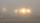The car started in fog at speed 30 km/h. After a 12-minute drive, the fog dissipated and the driver drove next 12 minutes distance 17 km. On the last 17 km long again the driving conditions deteriorated and the driver drove the speed of 51 km/h. a) Calcul
• Copper Cu wireCopper wire with a diameter of 1 mm and a weight of 350 g is wound on a spool. Calculate its length if the copper density is p = 8.9 g/cm cubic.
• Train delay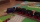Due to a breakdown, the train lost 16 minutes of standing on the track behind Brno. He "eliminated" this delay so that after the start, the 80 km long section went at a speed 10 km/h higher than originally planned. What speed was it and what was it suppos
• The copper wireThe copper wire bundle with a diameter of 2.8mm has a weight of 5kg. How many meters of wire is bundled if 1m3 of copper weighs 8930kg?
• Electric workCalculate the work done by the electric forces passing the current of 0.2 A through the bulb in 10 minutes if the bulb is connected to a 230 V power supply.
• Candles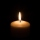Before Christmas, Eva bought two cylindrical candles - red and green. Red was 1 cm longer than green. She lit a red candle on Christmas Day at 5:30 p. M. , lit a green candle at 7:00 p. M. , and left them both on fire until they burned. At 9:30 p. M. , bo
• Two resistorsTwo resistors 20 Ω and 60 Ω are connected in series and an external voltage of 400 V is connected to them. What are the electrical voltages on the respective resistors? Please comment!
• Lowest voltage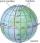Three resistors with resistors R1 = 10 kΩ, R2 = 20 kΩ, R3 = 30 kΩ are connected in series and an external voltage U = 30 V is connected to them. On which resistor is the lowest voltage?
• TransformerSolve the textbook problems - transformer: a) N1 = 40, N2 = 80, U2 = 80 V, U1 =? b) N1 = 400, U1 = 200 V, U2 = 50 V, N2 =?
• Filament of bulbThe filament of bulb has a 1 ohm resistivity and is connected to a voltage 220 V. How much electric charge will pass through the fiber when the electric current passes for 10 seconds?
• Coil as a girl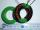The electrical resistance of the copper wire coil is 2.0 ohms. What current runs through the coil when the voltage between the terminals is 3.0 V?
• Resistance of the resistorThe resistor terminals have a voltage of 20 V and a current of 5 mA is passed through. What is the resistance of the resistor?
• ResistanceDetermine the resistance of the bulb with current 200 mA and is in regular lamp (230V).
• On the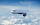On the map of Europe made at a scale of 1: 4000000, the distance between Bratislava and Paris is 28 cm. At what time an airplane flying at 800 km/h will fly this journey?
• The coal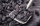The coal stock would be enough to heat a larger room for 12 weeks, a smaller one for 18 weeks. It was heated for four weeks in both rooms, then only in a smaller one. How long was the coal stock enough?
• Closed circuitIn a closed circuit, there is a voltage source with U1 = 12 V and with an internal resistance R1 = 0.2 Ω. The external resistance is R2 = 19.8 Ω. Determine the electric current and terminal voltage.
• Cooker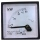A current of 2A passes through the immersion cooker at a voltage of 230V. What work do the electric field forces in 2 minutes?